Homework Help Question & Answers

# Advanced mechanics of solids

A state of plane strain exists at a point in a member with the following components

$$\epsilon_{x x}=-2000 \mu \quad \epsilon_{y y}=400 \mu \quad \epsilon_{x y}=-900 \mu$$

Determine the principal strain in (x,y) plane and orientation of rectangular element Determine the maximum shear strain in $$(\mathrm{x}, \mathrm{y})$$ and orientation of rectangular element.

The question marks are 𝜖𝑥𝑥, 𝜖𝑦𝑦 and 𝜖𝑥𝑦
Crystal888

#### Homework Answers

Answer #1 ✔ Recommended Answer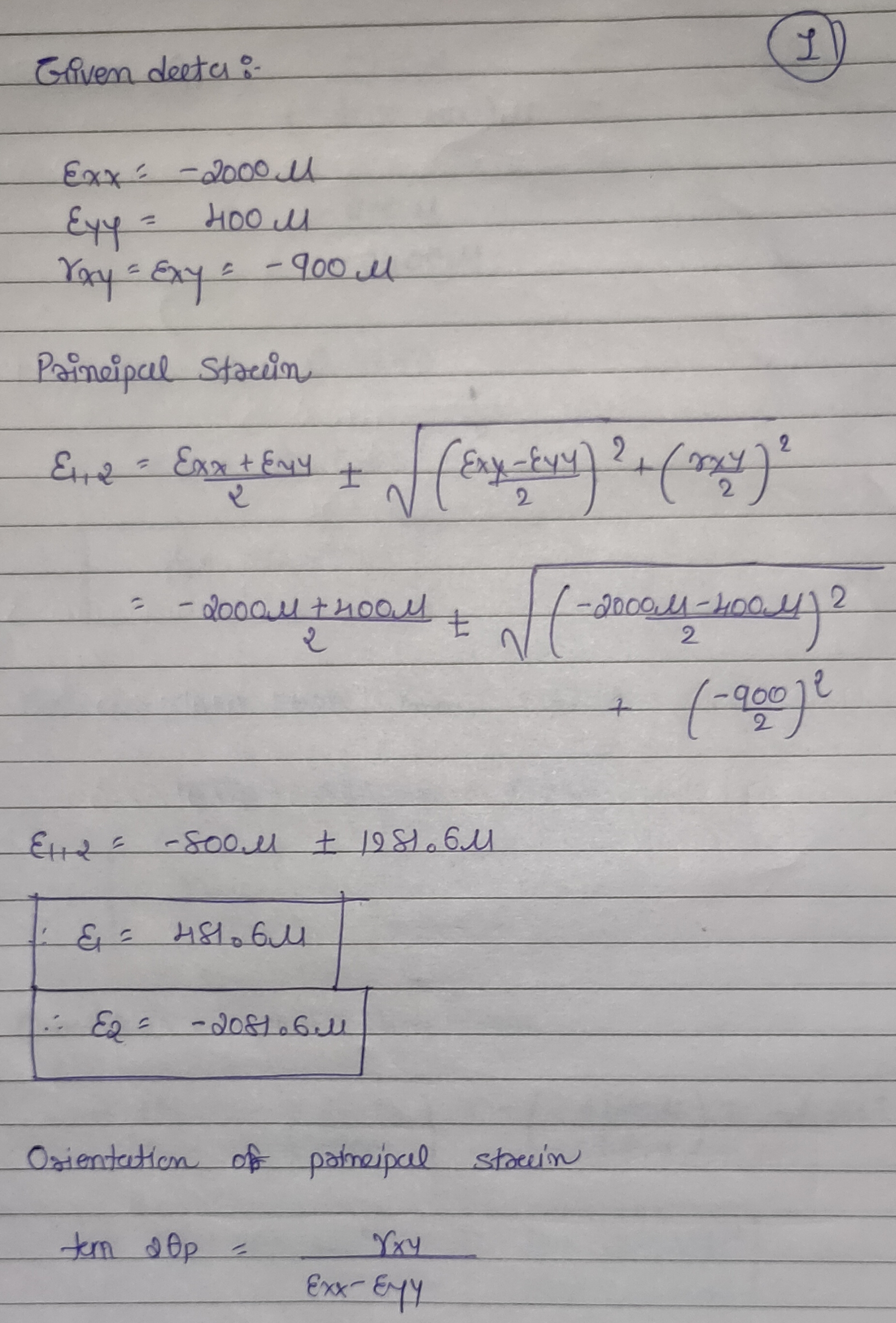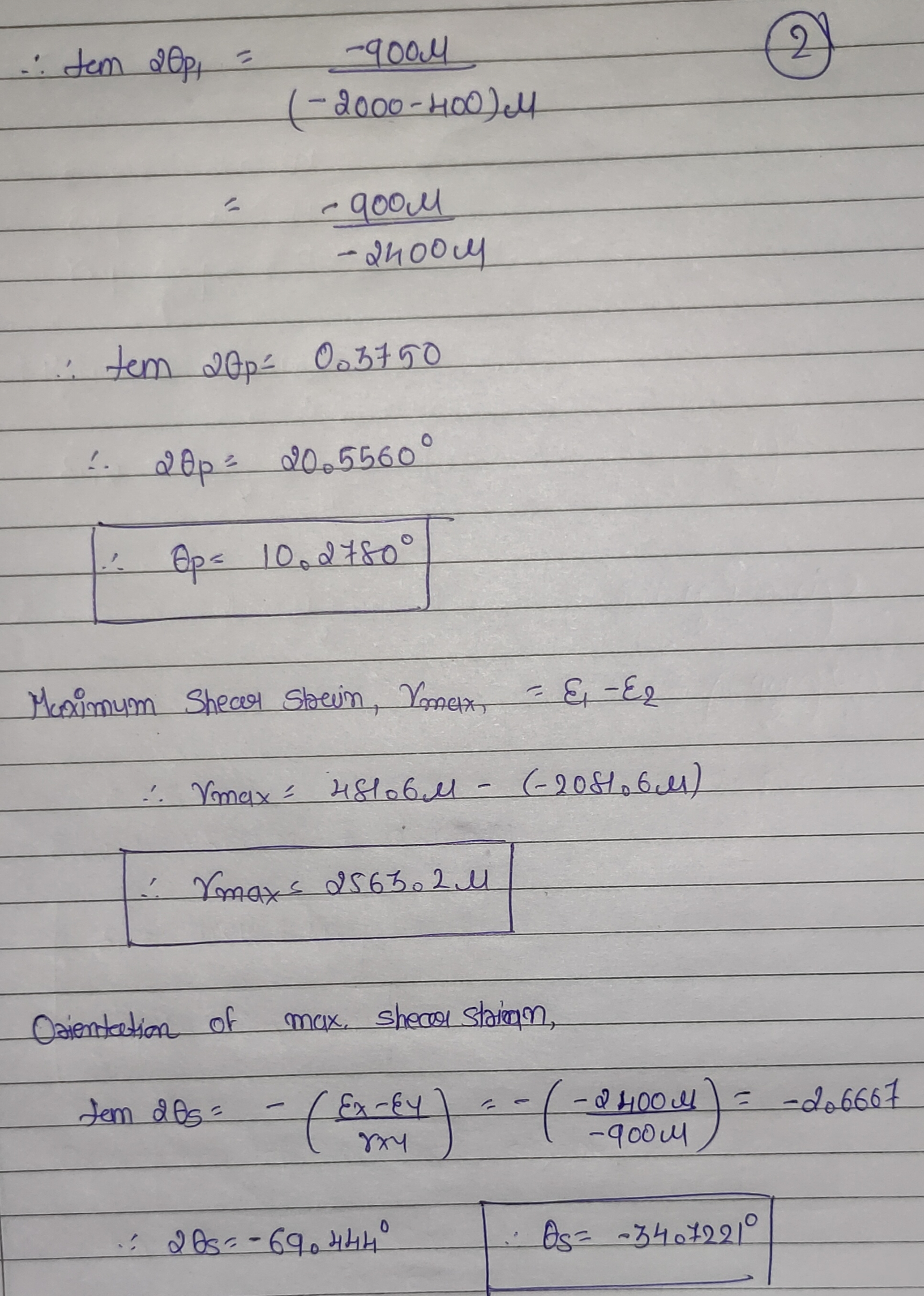Add a comment
Know the answer?
Your Answer:

#### Post as a guest

Your Name:

What's your source?

#### Earn Coin

Coins can be redeemed for fabulous gifts.

Similar Homework Help Questions
• ### Example: 1.2 A wrench is made of steel (E=210 GPa, v=0.3) with elastic constants of strain...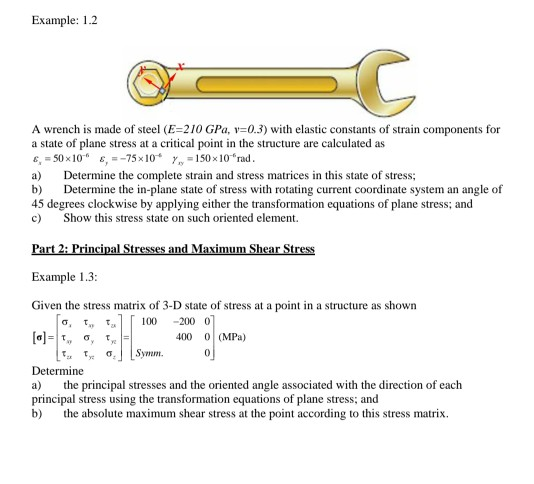Example: 1.2 A wrench is made of steel (E=210 GPa, v=0.3) with elastic constants of strain components for a state of plane stress at a critical point in the structure are calculated as 6, 50 x 10 = -75x10* 1 = 150 x 10"rad. a) Determine the complete strain and stress matrices in this state of stress, b) Determine the in-plane state of stress with rotating current coordinate system an angle of 45 degrees clockwise by applying either the transformation...

• ### Asap 1. The bracket is made of steel (Young's modulus 200 GPa; Poisson's ratio 0.3). When the force P is applied to the bracket, the gages in the strain rosette at point A have the followi...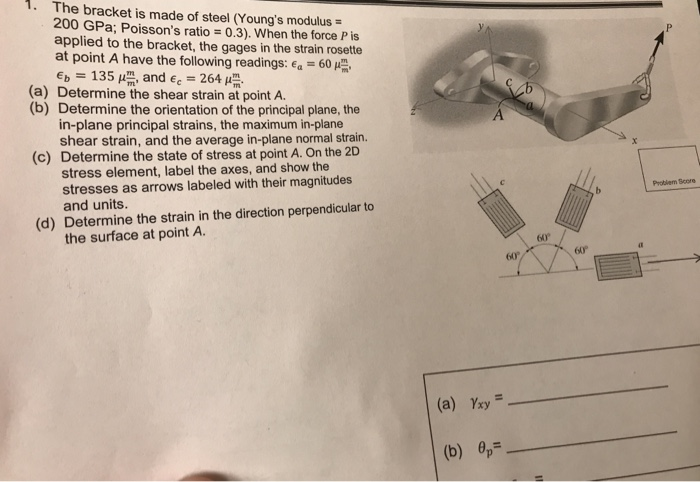Asap 1. The bracket is made of steel (Young's modulus 200 GPa; Poisson's ratio 0.3). When the force P is applied to the bracket, the gages in the strain rosette at point A have the following readings: E.-60 μ . Ep 135 μ l, and E.-264 μ (a) Determine the shear strain at point A. (b) Determine the orientation of the principal plane, the in-plane principal strains, the maximum in-plane shear strain, and the average in-plane normal strain. Determine the...

• ### Part C - Average normal strain in the differential element of material Determine the average normal...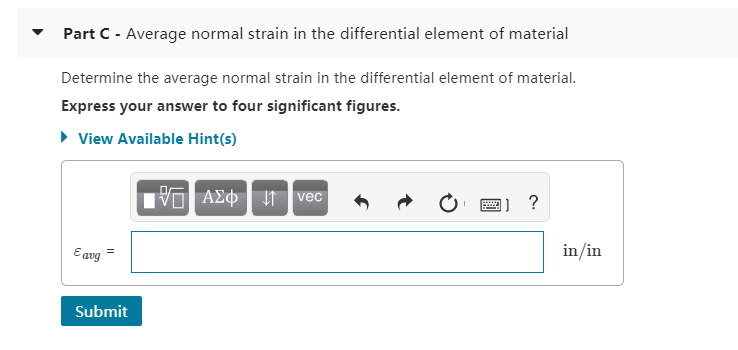Part C - Average normal strain in the differential element of material Determine the average normal strain in the differential element of material. Express your answer to four significant figures. View Available Hint(s) IPO AQ 1 vec R o 2 ? Eaug in/in Submit Part B - Maximum in-plane shear strain and its orientation Determine the magnitude of the maximum in-plane shear strain, marame, and its orientation relative to the differential element of material. Express your answers to four significant...

• ### 18- The slare of strain at the paint an the eaur ooth components of &#850(10°), ε,-480(10-6),...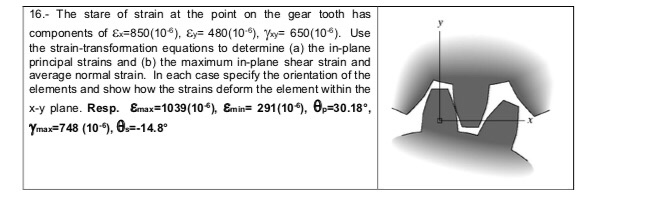18- The slare of strain at the paint an the eaur ooth components of &#850(10°), ε,-480(10-6), γ,-650(106). Use the strain-transfomation equations to determine (a) the in-plane principal strains and (b) the maximum in-plane shear strain and average normal strain. In each case specify the orientation of the elements and show how the strains deform the element within the x-y plane. Resp. &nax-1039(10-6), &nm-291 (109, θ-30.18", Ymax 748 (10,8148

• ### The strain rosette shown was used to obtain normal strain data at a point on the free surface of a machine component. Given the values εa= -185 με, εb= -150 με, εc= 55 με, E = 10,800 ksi, and v = 0.33...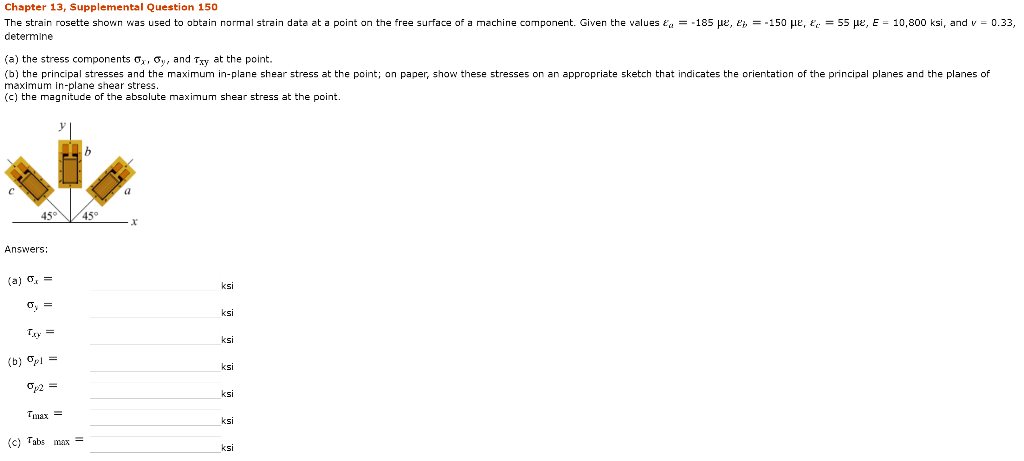The strain rosette shown was used to obtain normal strain data at a point on the free surface of a machine component. Given the values εa= -185 με, εb= -150 με, εc= 55 με, E = 10,800 ksi, and v = 0.33, determine (a) the stress components σx, σy, and τxy at the point. (b) the principal stresses and the maximum in-plane shear stress at the point; on paper, show these stresses on an appropriate sketch that indicates the orientation...

• ### The state of stress at a point on a body is given by the following stress...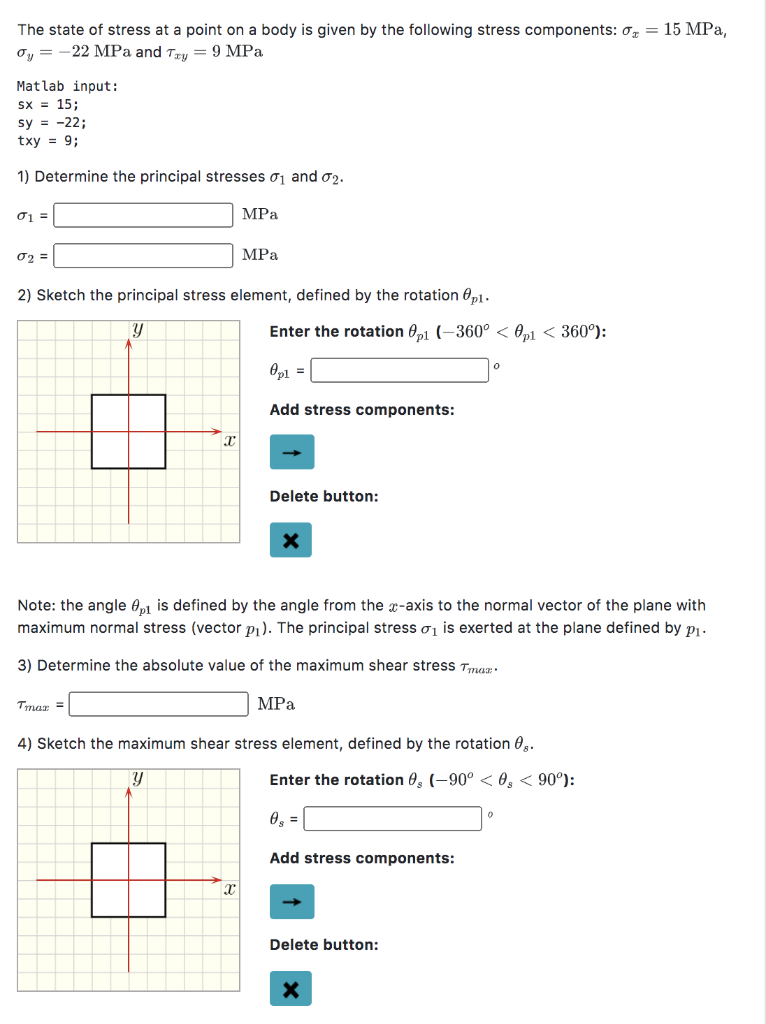The state of stress at a point on a body is given by the following stress components: 0 = 15 MPa, Oy = -22 MPa and Try = 9 MPa Matlab input: sx = 15; sy = -22; txy = 9; 1) Determine the principal stresses 01 and 02. 1 = MPa 02= MPa 2) Sketch the principal stress element, defined by the rotation @pl. y Enter the rotation @pi (-360º < Opl < 360°): Opl = Add stress components:...

• ### 23 Mohrs circle The state of plane stress at a point is represented by the element...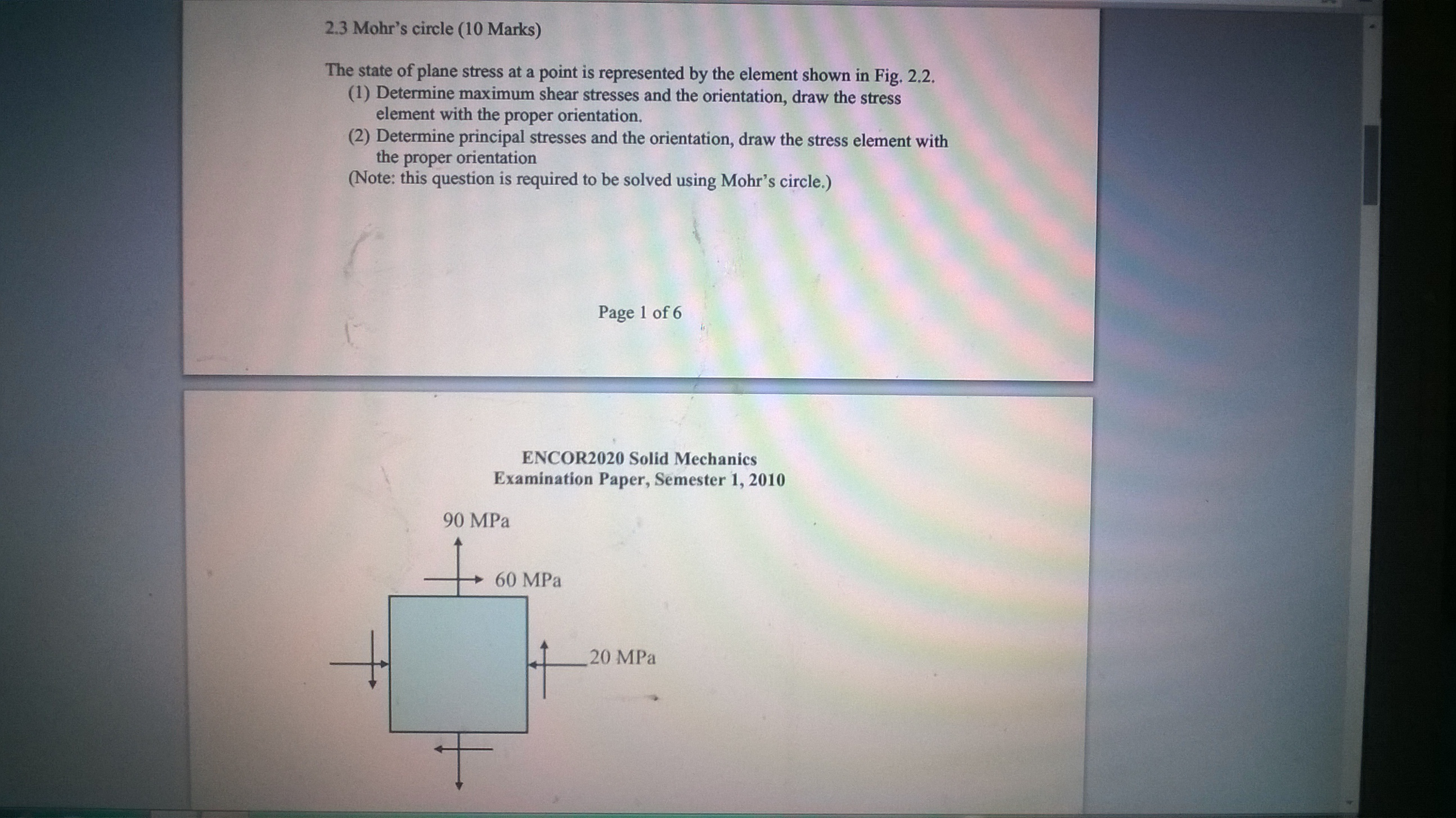23 Mohrs circle The state of plane stress at a point is represented by the element shown in Fig. 2.2. Determine maximum shear stresses and the orientation, draw the stress element with the proper orientation. Determine principal stresses and the orientation, draw the stress element with the proper orientation (Note: this question is required to be solved using Mohrs circle.) Mohrs circle The state of plane stress at a point is represented by the element shown in Fig. 2.2. Determine...

• ### When working through the equations, please thoroughly specify all the elements within each equation without skipping...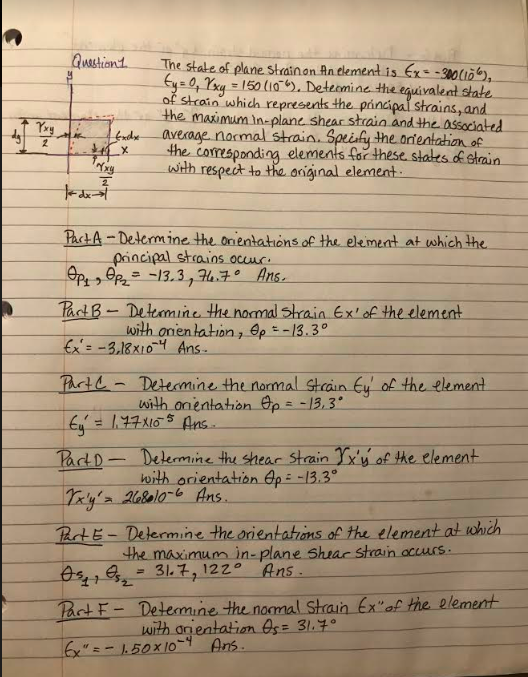When working through the equations, please thoroughly specify all the elements within each equation without skipping calculations. I need to know what goes inside the formulas and how they are derived to for each equation. I need it broken down as simple as possible as if teaching a 3rd grader. Thank you for your help! Question 1. The state of plane strain on An element is Ex-300010), tye 0, Yoxy = 150 (10) Determine the equivalent state of strain which...

• ### The state of stress at a point is shown on the element. Determine (a) the stress components acting on the inclined plan...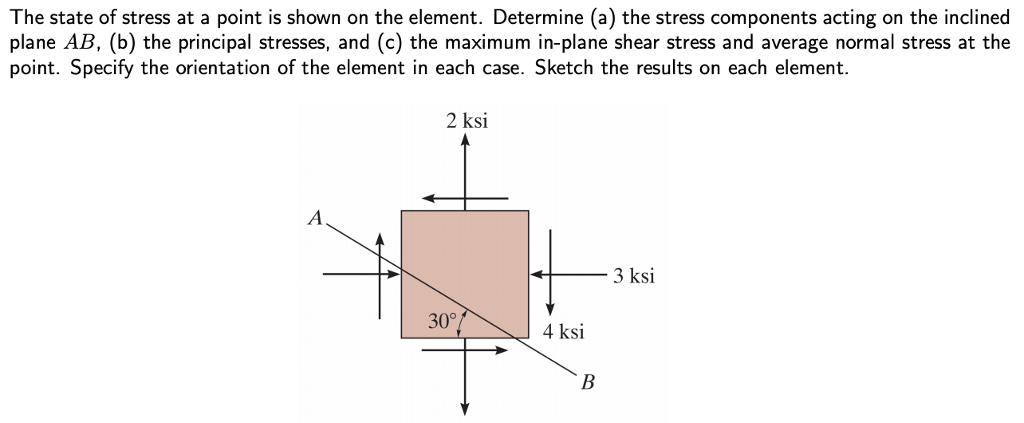The state of stress at a point is shown on the element. Determine (a) the stress components acting on the inclined plane AB, (b) the principal stresses, and (c) the maximum in-plane shear stress and average normal stress at the point. Specify the orientation of the element in each case. Sketch the results on each element. 2 ksi 3 ksi 30° 4 ksi

Free Homework App

Scan Your Homework
to Get Instant Free Answers
Need Online Homework Help?

Get Answers For Free
Most questions answered within 3 hours.